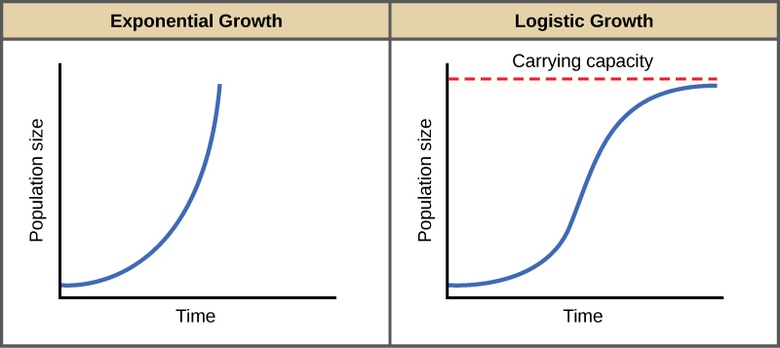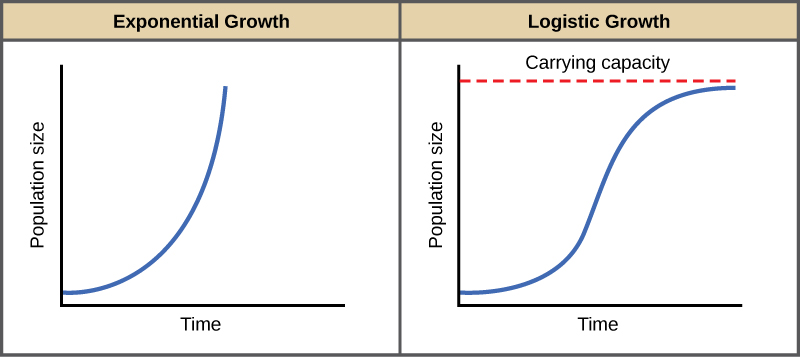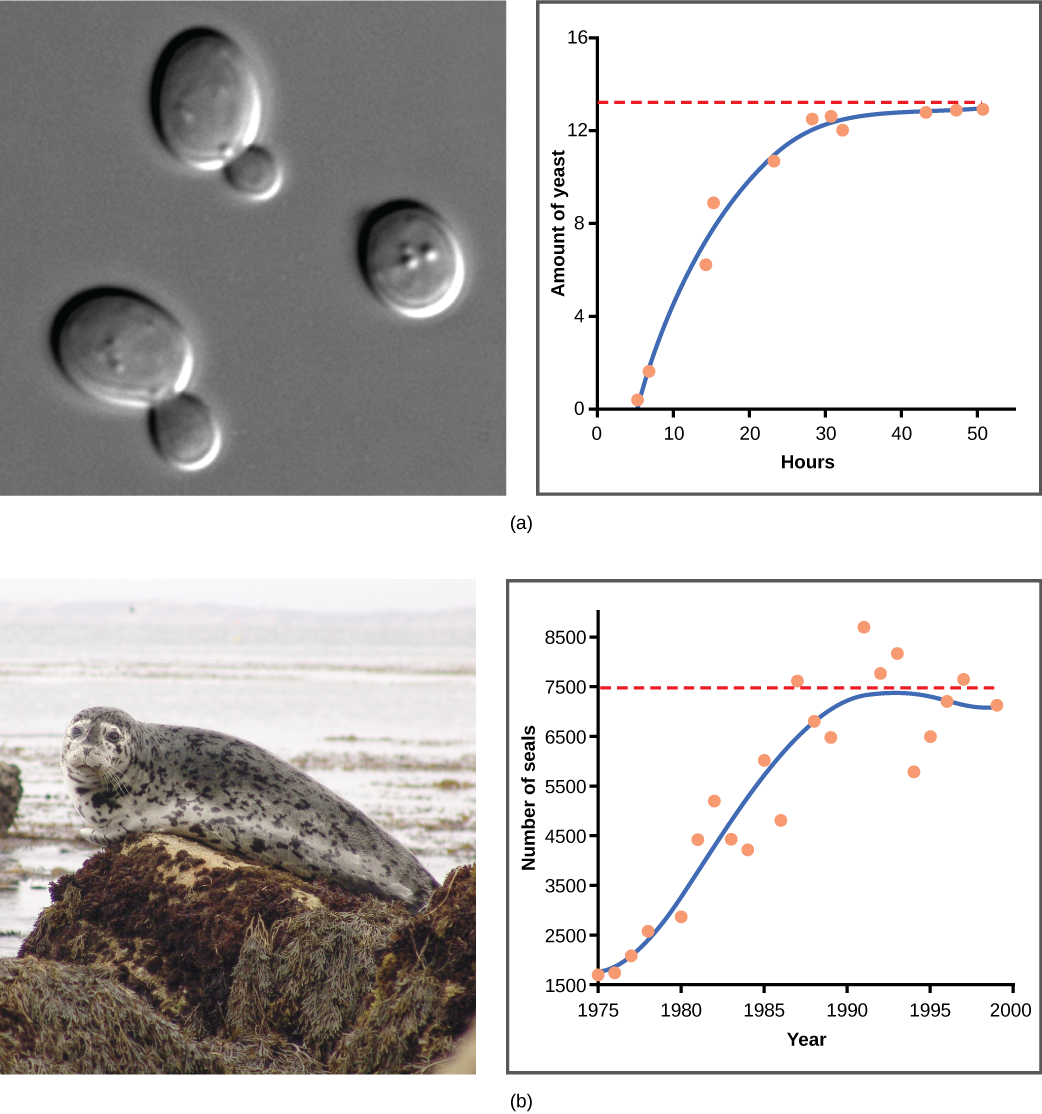Subject:
Applied Science, Life Science, Biology
Material Type:
Module
Level:
Community College / Lower Division, College / Upper Division
Provider:
Rice University
Tags:
Biotic Potential, Birth Rate, Carrying Capacity, Death Rate, Environmental Adaptation, Exponential Growth, Interspecific Competition, Intraspecific Competition, J-shaped Growth Curve, Logistic Growth, Population Ecology, S-shaped Growth Curve, Zero Population Growth
Language:
English

# Environmental Limits to Population Growth## Overview

By the end of this section, you will be able to:

• Explain the characteristics of and differences between exponential and logistic growth patterns
• Give examples of exponential and logistic growth in natural populations
• Describe how natural selection and environmental adaptation led to the evolution of particular life history patterns

Although life histories describe the way many characteristics of a population (such as their age structure) change over time in a general way, population ecologists make use of a variety of methods to model population dynamics mathematically. These more precise models can then be used to accurately describe changes occurring in a population and better predict future changes. Certain models that have been accepted for decades are now being modified or even abandoned due to their lack of predictive ability, and scholars strive to create effective new models.

# Exponential Growth

Charles Darwin, in his theory of natural selection, was greatly influenced by the English clergyman Thomas Malthus. Malthus published a book in 1798 stating that populations with unlimited natural resources grow very rapidly, and then population growth decreases as resources become depleted. This accelerating pattern of increasing population size is called exponential growth.

The best example of exponential growth is seen in bacteria. Bacteria are prokaryotes that reproduce by prokaryotic fission. This division takes about an hour for many bacterial species. If 1000 bacteria are placed in a large flask with an unlimited supply of nutrients (so the nutrients will not become depleted), after an hour, there is one round of division and each organism divides, resulting in 2000 organisms—an increase of 1000. In another hour, each of the 2000 organisms will double, producing 4000, an increase of 2000 organisms. After the third hour, there should be 8000 bacteria in the flask, an increase of 4000 organisms. The important concept of exponential growth is that the population growth rate—the number of organisms added in each reproductive generation—is accelerating; that is, it is increasing at a greater and greater rate. After 1 day and 24 of these cycles, the population would have increased from 1000 to more than 16 billion. When the population size, N, is plotted over time, a J-shaped growth curve is produced (Figure).

The bacteria example is not representative of the real world where resources are limited. Furthermore, some bacteria will die during the experiment and thus not reproduce, lowering the growth rate. Therefore, when calculating the growth rate of a population, the death rate (D) (number organisms that die during a particular time interval) is subtracted from the birth rate (B) (number organisms that are born during that interval). This is shown in the following formula:

The birth rate is usually expressed on a per capita (for each individual) basis. Thus, B (birth rate) = bN (the per capita birth rate “b” multiplied by the number of individuals “N”) and D (death rate) =dN (the per capita death rate “d” multiplied by the number of individuals “N”). Additionally, ecologists are interested in the population at a particular point in time, an infinitely small time interval. For this reason, the terminology of differential calculus is used to obtain the “instantaneous” growth rate, replacing the change in number and time with an instant-specific measurement of number and time.

Notice that the “d” associated with the first term refers to the derivative (as the term is used in calculus) and is different from the death rate, also called “d.” The difference between birth and death rates is further simplified by substituting the term “r” (intrinsic rate of increase) for the relationship between birth and death rates:

The value “r” can be positive, meaning the population is increasing in size; or negative, meaning the population is decreasing in size; or zero, where the population’s size is unchanging, a condition known as zero population growth. A further refinement of the formula recognizes that different species have inherent differences in their intrinsic rate of increase (often thought of as the potential for reproduction), even under ideal conditions. Obviously, a bacterium can reproduce more rapidly and have a higher intrinsic rate of growth than a human. The maximal growth rate for a species is its biotic potential, or rmax, thus changing the equation to:When resources are unlimited, populations exhibit exponential growth, resulting in a J-shaped curve. When resources are limited, populations exhibit logistic growth. In logistic growth, population expansion decreases as resources become scarce, and it levels off when the carrying capacity of the environment is reached, resulting in an S-shaped curve.

# Logistic Growth

Exponential growth is possible only when infinite natural resources are available; this is not the case in the real world. Charles Darwin recognized this fact in his description of the “struggle for existence,” which states that individuals will compete (with members of their own or other species) for limited resources. The successful ones will survive to pass on their own characteristics and traits (which we know now are transferred by genes) to the next generation at a greater rate (natural selection). To model the reality of limited resources, population ecologists developed the logistic growth model.

## Carrying Capacity and the Logistic Model

In the real world, with its limited resources, exponential growth cannot continue indefinitely. Exponential growth may occur in environments where there are few individuals and plentiful resources, but when the number of individuals gets large enough, resources will be depleted, slowing the growth rate. Eventually, the growth rate will plateau or level off (Figure). This population size, which represents the maximum population size that a particular environment can support, is called the carrying capacity, or K.

The formula we use to calculate logistic growth adds the carrying capacity as a moderating force in the growth rate. The expression “KN” is indicative of how many individuals may be added to a population at a given stage, and “KN” divided by “K” is the fraction of the carrying capacity available for further growth. Thus, the exponential growth model is restricted by this factor to generate the logistic growth equation:

Notice that when N is very small, (K-N)/K becomes close to K/K or 1, and the right side of the equation reduces to rmaxN, which means the population is growing exponentially and is not influenced by carrying capacity. On the other hand, when N is large, (K-N)/K come close to zero, which means that population growth will be slowed greatly or even stopped. Thus, population growth is greatly slowed in large populations by the carrying capacity K. This model also allows for the population of a negative population growth, or a population decline. This occurs when the number of individuals in the population exceeds the carrying capacity (because the value of (K-N)/K is negative).

A graph of this equation yields an S-shaped curve (Figure), and it is a more realistic model of population growth than exponential growth. There are three different sections to an S-shaped curve. Initially, growth is exponential because there are few individuals and ample resources available. Then, as resources begin to become limited, the growth rate decreases. Finally, growth levels off at the carrying capacity of the environment, with little change in population size over time.

## Role of Intraspecific Competition

The logistic model assumes that every individual within a population will have equal access to resources and, thus, an equal chance for survival. For plants, the amount of water, sunlight, nutrients, and the space to grow are the important resources, whereas in animals, important resources include food, water, shelter, nesting space, and mates.

In the real world, phenotypic variation among individuals within a population means that some individuals will be better adapted to their environment than others. The resulting competition between population members of the same species for resources is termed intraspecific competition (intra- = “within”; -specific = “species”). Intraspecific competition for resources may not affect populations that are well below their carrying capacity—resources are plentiful and all individuals can obtain what they need. However, as population size increases, this competition intensifies. In addition, the accumulation of waste products can reduce an environment’s carrying capacity.

## Examples of Logistic Growth

Yeast, a microscopic fungus used to make bread and alcoholic beverages, exhibits the classical S-shaped curve when grown in a test tube (Figurea). Its growth levels off as the population depletes the nutrients that are necessary for its growth. In the real world, however, there are variations to this idealized curve. Examples in wild populations include sheep and harbor seals (Figureb). In both examples, the population size exceeds the carrying capacity for short periods of time and then falls below the carrying capacity afterwards. This fluctuation in population size continues to occur as the population oscillates around its carrying capacity. Still, even with this oscillation, the logistic model is confirmed.

### Art Connection(a) Yeast grown in ideal conditions in a test tube show a classical S-shaped logistic growth curve, whereas (b) a natural population of seals shows real-world fluctuation.

If the major food source of the seals declines due to pollution or overfishing, which of the following would likely occur?

1. The carrying capacity of seals would decrease, as would the seal population.
2. The carrying capacity of seals would decrease, but the seal population would remain the same.
3. The number of seal deaths would increase but the number of births would also increase, so the population size would remain the same.
4. The carrying capacity of seals would remain the same, but the population of seals would decrease.

# Section Summary

Populations with unlimited resources grow exponentially, with an accelerating growth rate. When resources become limiting, populations follow a logistic growth curve. The population of a species will level off at the carrying capacity of its environment.

# Art Connections

Figureb If the major food source of the seals declines due to pollution or overfishing, which of the following would likely occur?

1. The carrying capacity of seals would decrease, as would the seal population.
2. The carrying capacity of seals would decrease, but the seal population would remain the same.
3. The number of seal deaths would increase but the number of births would also increase, so the population size would remain the same.
4. The carrying capacity of seals would remain the same, but the population of seals would decrease.

Figureb A

# Review Questions

Species with limited resources usually exhibit a(n) ________ growth curve.

1. logistic
2. logical
3. experimental
4. exponential

## Hint:

A

The maximum rate of increased characteristic of a species is called its ________.

1. limit
2. carrying capacity
3. biotic potential
4. exponential growth pattern

## Hint:

C

The population size of a species capable of being supported by the environment is called its ________.

1. limit
2. carrying capacity
3. biotic potential
4. logistic growth pattern

B

# Free Response

Describe the rate of population growth that would be expected at various parts of the S-shaped curve of logistic growth.

## Hint:

In the first part of the curve, when few individuals of the species are present and resources are plentiful, growth is exponential, similar to a J-shaped curve. Later, growth slows due to the species using up resources. Finally, the population levels off at the carrying capacity of the environment, and it is relatively stable over time.